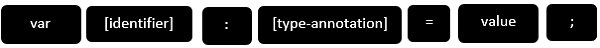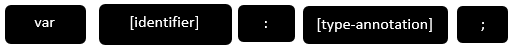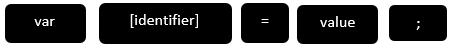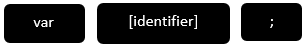# TypeScript - Variables

A variable, by definition, is “a named space in the memory” that stores values. In other words, it acts as a container for values in a program. TypeScript variables must follow the JavaScript naming rules −

• Variable names can contain alphabets and numeric digits.

• They cannot contain spaces and special characters, except the underscore (_) and the dollar (\$) sign.

• Variable names cannot begin with a digit.

A variable must be declared before it is used. Use the var keyword to declare variables.

## Variable Declaration in TypeScript

The type syntax for declaring a variable in TypeScript is to include a colon (:) after the variable name, followed by its type. Just as in JavaScript, we use the var keyword to declare a variable.

When you declare a variable, you have four options −

• Declare its type and value in one statement.• Declare its type but no value. In this case, the variable will be set to undefined.• Declare its value but no type. The variable type will be set to the data type of the assigned value.• Declare neither value not type. In this case, the data type of the variable will be any and will be initialized to undefined.The following table illustrates the valid syntax for variable declaration as discussed above −

S.No. Variable Declaration Syntax & Description
1.

var name:string = ”mary”

The variable stores a value of type string

2.

var name:string;

The variable is a string variable. The variable’s value is set to undefined by default

3.

var name = ”mary”

The variable’s type is inferred from the data type of the value. Here, the variable is of the type string

4.

var name;

The variable’s data type is any. Its value is set to undefined by default.

### Example: Variables in TypeScript

```var name:string = "John";
var score1:number = 50;
var score2:number = 42.50
var sum = score1 + score2
console.log("name"+name)
console.log("first score: "+score1)
console.log("second score: "+score2)
console.log("sum of the scores: "+sum)
```

On compiling, it will generate following JavaScript code.

```//Generated by typescript 1.8.10
var name = "John";
var score1 = 50;
var score2 = 42.50;
var sum = score1 + score2;
console.log("name" + name);
console.log("first score: " + score1);
console.log("second score : " + score2);
console.log("sum of the scores: " + sum);
```

The output of the above program is given below −

```name:John
first score:50
second score:42.50
sum of the scores:92.50
```

The TypeScript compiler will generate errors, if we attempt to assign a value to a variable that is not of the same type. Hence, TypeScript follows Strong Typing. The Strong typing syntax ensures that the types specified on either side of the assignment operator (=) are the same. This is why the following code will result in a compilation error −

```var num:number = "hello"     // will result in a compilation error
```

## Type Assertion in TypeScript

TypeScript allows changing a variable from one type to another. TypeScript refers to this process as Type Assertion. The syntax is to put the target type between < > symbols and place it in front of the variable or expression. The following example explains this concept −

### Example

```var str = '1'
var str2:number = <number> <any> str   //str is now of type number
console.log(typeof(str2))
```

If you hover the mouse pointer over the type assertion statement in Visual Studio Code, it displays the change in the variable’s data type. Basically it allows the assertion from type S to T succeed if either S is a subtype of T or T is a subtype of S.

The reason why it's not called "type casting" is that casting generally implies some sort of runtime support while, “type assertions” are purely a compile time construct and a way for you to provide hints to the compiler on how you want your code to be analyzed.

On compiling, it will generate following JavaScript code.

```"use strict";
var str = '1';
var str2 = str; //str is now of type number
console.log(typeof (str2));
```

It will produce the following output −

```string
```

## Inferred Typing in TypeScript

Given the fact that, Typescript is strongly typed, this feature is optional. TypeScript also encourages dynamic typing of variables. This means that, TypeScript encourages declaring a variable without a type. In such cases, the compiler will determine the type of the variable on the basis of the value assigned to it. TypeScript will find the first usage of the variable within the code, determine the type to which it has been initially set and then assume the same type for this variable in the rest of your code block.

The same is explained in the following code snippet −

### Example: Inferred Typing

```var num = 2;    // data type inferred as  number
console.log("value of num "+num);
num = "12";
console.log(num);
```

In the above code snippet −

• The code declares a variable and sets its value to 2. Note that the variable declaration doesn’t specify the data type. Hence, the program uses inferred typing to determine the data type of the variable, i.e., it assigns the type of the first value that the variable is set to. In this case, num is set to the type number.

• When the code tries to set the variable’s value to string. The compiler throws an error as the variable’s type is already set to number.

It will produce the following output −

```error TS2011: Cannot convert 'string' to 'number'.
```

## TypeScript Variable Scope

The scope of a variable specifies where the variable is defined. The availability of a variable within a program is determined by its scope. TypeScript variables can be of the following scopes −

• Global Scope − Global variables are declared outside the programming constructs. These variables can be accessed from anywhere within your code.

• Class Scope − These variables are also called fields. Fields or class variables are declared within the class but outside the methods. These variables can be accessed using the object of the class. Fields can also be static. Static fields can be accessed using the class name.

• Local Scope − Local variables, as the name suggests, are declared within the constructs like methods, loops etc. Local variables are accessible only within the construct where they are declared.

The following example illustrates variable scopes in TypeScript.

### Example: Variable Scope

```var global_num = 12          //global variable
class Numbers {
num_val = 13;             //class variable
static sval = 10;         //static field

storeNum():void {
var local_num = 14;    //local variable
}
}
console.log("Global num: "+global_num)
console.log(Numbers.sval)   //static variable
var obj = new Numbers();
console.log("Global num: "+obj.num_val)
```

On transpiling, the following JavaScript code is generated −

```var global_num = 12;              //global variable
var Numbers = (function () {
function Numbers() {
this.num_val = 13;          //class variable
}
Numbers.prototype.storeNum = function () {
var local_num = 14;        //local variable
};
Numbers.sval = 10;            //static field
return Numbers;
}());

console.log("Global num: " + global_num);
console.log(Numbers.sval);       //static variable

var obj = new Numbers();
console.log("Global num: " + obj.num_val);
```

It will produce the following output −

```Global num: 12
10
Global num: 13
```

If you try accessing the local variable outside the method, it results in a compilation error.

```error TS2095: Could not find symbol 'local_num'.
```